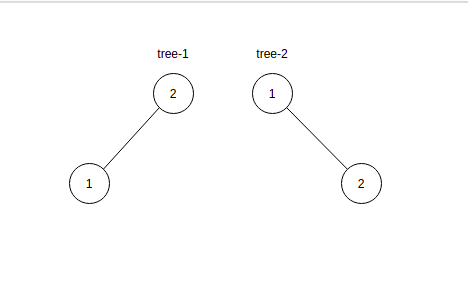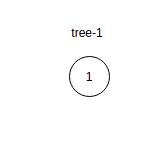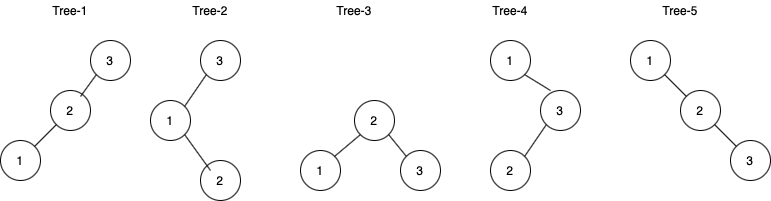2

Count unique BSTs.

Difficulty: HARD
Avg. time to solve
15 min
Success Rate
85%

Problem Statement

Two binary search trees are different if there is at least one node during traversal which is different in values or present in one tree but not present in another tree.

Note:
The answer can be large, hence the return answer % ‘MOD’, where ‘MOD’ is a large prime number (10^9 + 7).
Input format :
The first line contains a single integer ‘T’ representing the number of test cases.

The first line of each test case will contain a single integer ‘NUM’ where ‘NUM’ represents the number of keys.
Output Format :
For each test case, print a single line containing the count of total structurally unique binary search trees.

The output of each test case will be printed in a separate line.
Note:
You don’t need to print anything; It has already been taken care of. Just implement the given function.
Constraints:
1 <= T <= 100
1 <= NUM <= 500

Time limit: 1 sec
2
2
1
2
1
Explanation of sample input 1:
In the first test case, 2 unique BST are possible.In the second test case, only 1 tree is possible.2
3
4
5
14
Explanation of sample input 2:
In the first test case, 5 unique BST are possible.In the second test case, similar to the above way, 14 unique BST are possible.Console